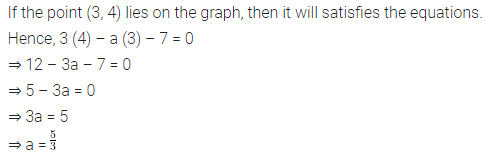# If the point (3,4) lies on the graph of the equation 3y – ax – 7, find the value of a

If the point (3,4) lies on the graph of the equation 3y – ax – 7, find the value of a.